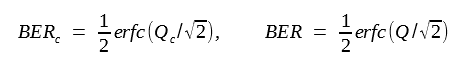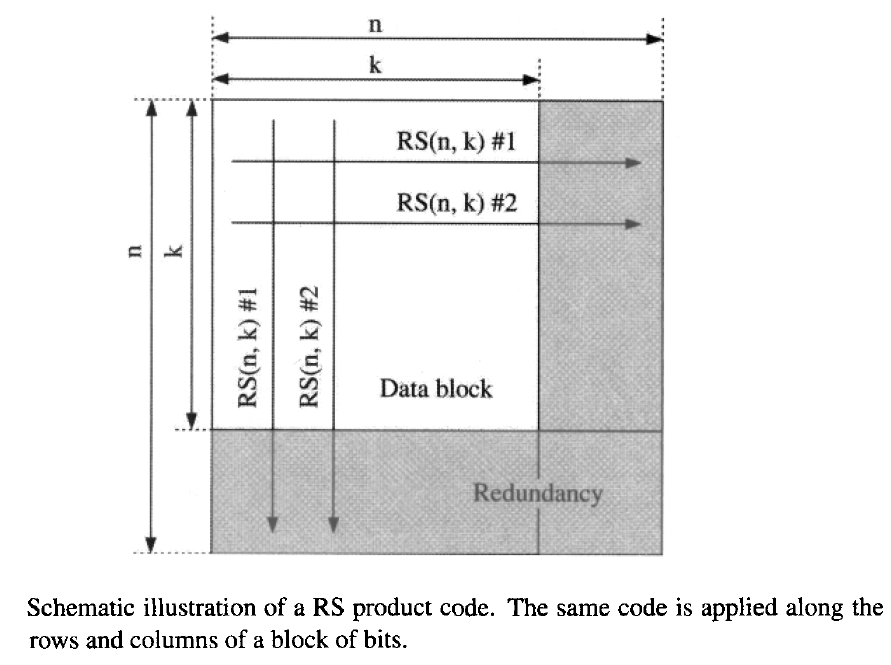# Forward Error Correction

As seen in the preceding tutorials, receiver sensitivity and the BER of a lightwave system are degraded by many factors that are not always controllable in practice. Depending on details of the system design and objectives, it is entirely possible that a specified BER cannot be achieved. Under such conditions, the use of an error-correction scheme remains the only viable alternative.

Error control is not a new concept and is employed widely in electrical systems dealing with the transfer of digital data from one device to another. The techniques used for controlling errors can be divided into two groups. In one group, errors are detected but not corrected. Rather, each packet of bits received with errors is retransmitted. This approach is suitable when data bits are transmitted in the form of packets (as is the case for the protocol used by the Internet) and they do not arrive at the destination in a synchronous fashion. In the other group, errors are detected as well as corrected at the receiver end without any retransmission of bits. This approach is referred to as forward error correction (FEC) and is best suited for lightwave systems operating with a synchronous protocol such as SONET or SDH.

Historically, lightwave systems did not employ FEC until the use of in-line optical amplifiers became common. The use of FEC accelerated with the advent of WDM technology. As early as 1996, FEC was employed for a WDM system designed to operate over more than 425 km without any in-line amplifier or regenerator. Since then, the FEC technique has been used in many WDM systems and is now considered almost routine.

#### 1. Error-Correcting Codes

The basic idea behind any error-control technique is to add extra bits to the signal at the transmitter end in a judicial manner using a suitable coding algorithm. A simple example is provided by the so-called parity bit that is added to the 7-bit ASCII code. In this example, the parity bit is chosen to be 0 or 1 depending on whether the number of 1 bits in the 7-bit sequence is even or odd. If a single bit is in error at the receiving end, an examination of the parity bit reveals the error.

The situation is somewhat different in the case of an optical bit stream, but the basic idea remains the same. An encoder within the transmitter adds additional control bits using a suitable code. At the receiver end, a decoder uses these control bits to detect errors, while correcting them simultaneously. How many errors can be corrected depends on the coding scheme employed. In general, more errors can be corrected by adding more control bits to the signal. Clearly, there is a limit to this process since the bit rate of the signal increases after the decoder. If Be is the effective bit rate after coding a signal at the bit rate B,  the FEC overhead associated with the error-correcting code is Be/B - 1. The concept of redundancy is also used for FEDC codes as the bits added by the coding scheme do not carry any information. Redundancy of a code is defined as ρ = 1 - B/Be.

Many different types of error-correcting codes have been developed, often classified under names such as linear, cyclic, Hamming, Reed-Solomon, convolutional, product, and turbo codes. Among these, Reed-Solomon (RS) codes have attracted most attention in the context of lightwave systems. An RS code is denoted as RS(n,k), where k is the size of a package of bits that is converted through coding into a larger packet with n bits. The value of n is chosen such that n = 2m - 1, where m is an integer. The RS code recommended by ITU for submarine applications uses m = 8 and is written as RS(255, 239). The FEC overhead for this code is only 6.7%. Many other RS codes can be used if a higher overhead is permitted. For example, the code RS(255, 207) has an overhead of 23.2% but it allows for more robust error control. The choice of the code depends on the level of improvement in the BER required for the system to operate reliably. It is common to quantify this improvement through the coding gain, a concept we discuss next.

#### 2. Coding Gain

Coding gain is a measure of the improvement in BER realized through FEC. Since BER is related to the Q factor, it is often expressed in terms of the equivalent value of Q that corresponds to the BER realized after the FEC decoder. The coding gain in decibel is defined as

Gc = 20 log10(Qc/Q)

where Qc and Q are related to the BERs obtained with and without FEC asThe factor of 20 appears in place of 10 because Q2 is traditionally used for expressing the Q factor in decibel units. As an example, if the FEC decoder improves the BER from its original value of 10-3 to 10-9, the value of Q increases from about 3 to 6, resulting in a coding gain of 6 dB. The coding gain is sometimes defined in terms of the SNR. The two definitions differ by a small amount of 10log10(Be/B).

As one would expect, the magnitude of coding gain increases with the FEC overhead (or redundancy). The dashed line in this figure shows this behaver.The coding gain is about 5.5 dB for 10% overhead and increases sublinearly, reaching only 8 dB even for a 50% overhead. It can be improved by concatenating two or more RS codes or by employing the RS product codes, but in all cases the coding gain begins to saturate as overhead increases. In the case of a RS product code, more than 6 dB of coding gain can be realized with only 5% overhead. The basic idea behind an RS product code is shown in the figure below.As seen there, a block of data with k2 bits is converted into n2 bits by applying the same RS(n,k) code both along the rows and columns. As a result, the overhead of n2/k2 - 1 for a RS product code is larger, but it also allows more error control.